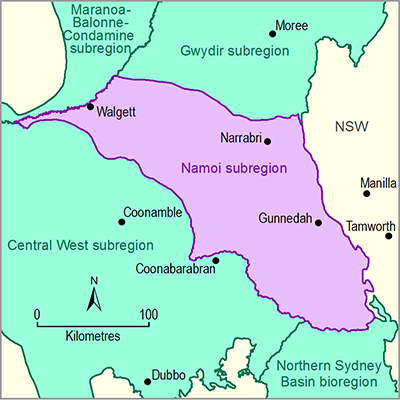# 2.6.1.6.1 Introduction

Page 30 of 41

Section 2.6.1.6 summarises the prediction results for nine hydrological response variables and for 54 surface water model nodes. The nine hydrological response variables for streamflow are:

• P01 – the daily flow rate at the 1st percentile (ML/day). This is typically reported as the maximum change due to additional coal resource development over the 90-year period (from 2013 to 2102).
• zero-flow days (ZFD) – the number of zero-flow days per year. This is typically reported as the maximum change due to additional coal resource development over the 90-year period (from 2013 to 2102).
• low-flow days (LFD) – the number of low-flow days per year. This is typically reported as the maximum change due to additional coal resource development over the 90-year period (from 2013 to 2102). The threshold for low-flow days is the 10th percentile from the simulated 90-year period (2013 to 2102)
• low-flow spells (LFS) – the number of low-flow spells per year (perennial streams only). This is typically reported as the maximum change due to additional coal resource development over the 90-year period (from 2013 to 2102). A spell is defined as a period of contiguous days of streamflow below the 10th percentile threshold
• length of low-flow spell (LLFS) – the length (days) of the longest low-flow spell each year. This is typically reported as the maximum change due to additional coal resource development over the 90-year period (from 2013 to 2102).
• P99 – the daily flow rate at the 99th percentile (ML/day). This is typically reported as the maximum change due to additional coal resource development over the 90-year period (from 2013 to 2102).
• high-flow days (FD) – the number of high-flow days per year. This is typically reported as the maximum change due to additional coal resource development over the 90-year period (from 2013 to 2102). The threshold for high-flow days is the 90th percentile from the simulated 90-year period (2013 to 2102). In some early products, this was referred to as ‘flood days’
• annual flow (AF) – the volume of water that discharges past a specific point in a stream in a year, commonly measured in GL/year. This is typically reported as the maximum change due to additional coal resource development over the 90-year period (from 2013 to 2102).
• interquartile range (IQR) – the interquartile range in daily flow (ML/day); that is, the difference between the daily flow rate at the 75th percentile and at the 25th percentile. This is typically reported as the maximum change due to additional coal resource development over the 90-year period (from 2013 to 2102).

These hydrological response variables were chosen to enable quantification of changes across the entire flow regime (see companion submethodology M06 (as listed in Table 1) for surface water modelling (Viney, 2016)). For each of these hydrological response variables a time series of annual values for the period 2013 to 2102 is constructed for each model node.

For each model node, 3000 sets of randomly selected parameter values were used to generate 3000 replicates of coal resource development impact. From these, the best 300 replicates for each hydrological response variable – as assessed by their ability to predict that hydrological response variable at the 28 sites where suitable observed data was available – were chosen for further analysis. The distributions of hydrological change from the resulting 300 samples are presented in boxplots in Section 2.6.1.6.2. The boxplots show the distributions over the 300 replicates of the maximum raw change (amax) in each metric between the baseline and coal resource development pathway (CRDP) predictions, the corresponding maximum percent change (pmax) and the year of maximum change (tmax). In general, the most meaningful diagnostic for the flux-based metrics (P01, P99, AF and IQR) will be pmax, while the most meaningful diagnostic for the frequency-based metrics (LFD, LFS, LLFS, ZFD and FD) will be amax.

It is important to recognise that the amax and pmax values give the largest annual departure between the baseline and CRDP predictions for the respective hydrological response variables. As such, amax and pmax represent extreme responses. They do not represent the magnitude of responses that would be expected to occur every year.

Last updated:
6 December 2018